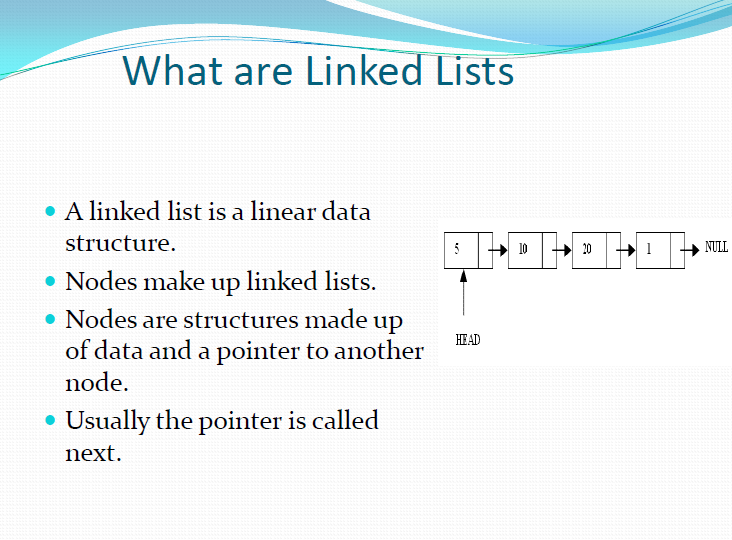Programming BooksA linked list is a linear data structure. There is nodes and link with each other. one node points to the next node and pointer called to other

• We use two are using two classes Node and List
• Declare both  Node class for the nodes
• in this example data: double-type data in this example
• in this example next: a pointer to the next node in the list
 class Node { public: double  data;  // data Node*  next;  // pointer to next };

There are two basic types of linked list

you can find the detail in BOOk